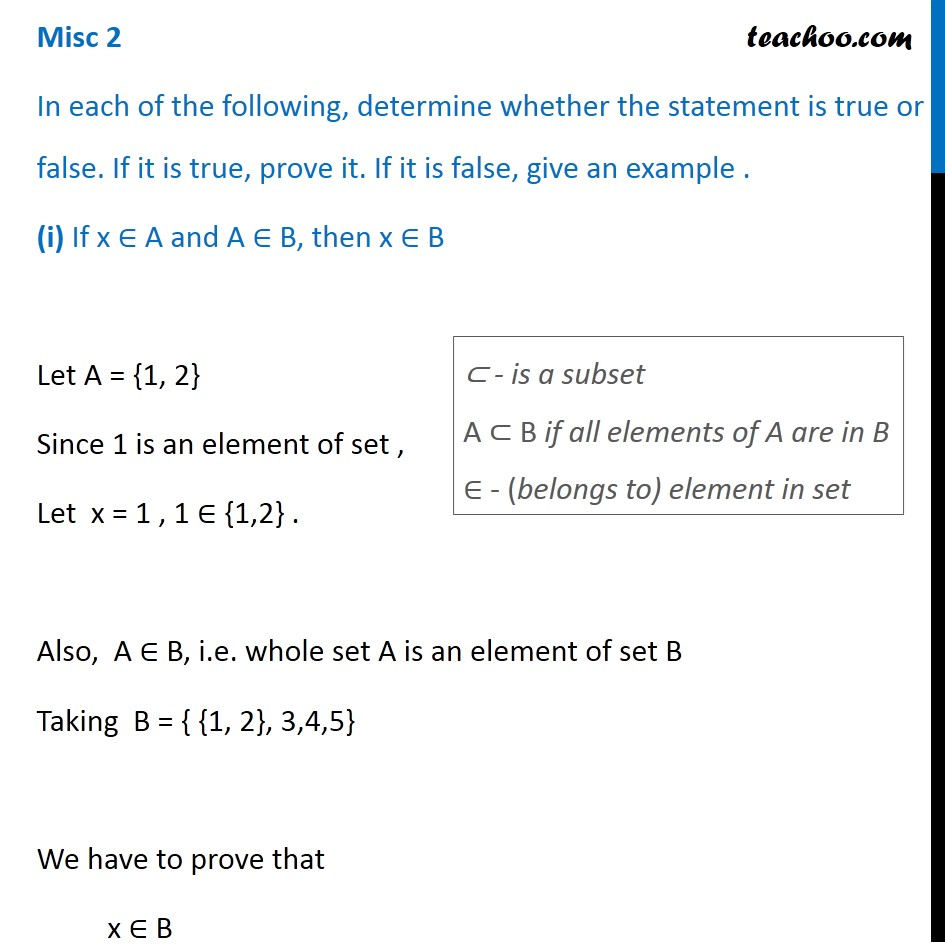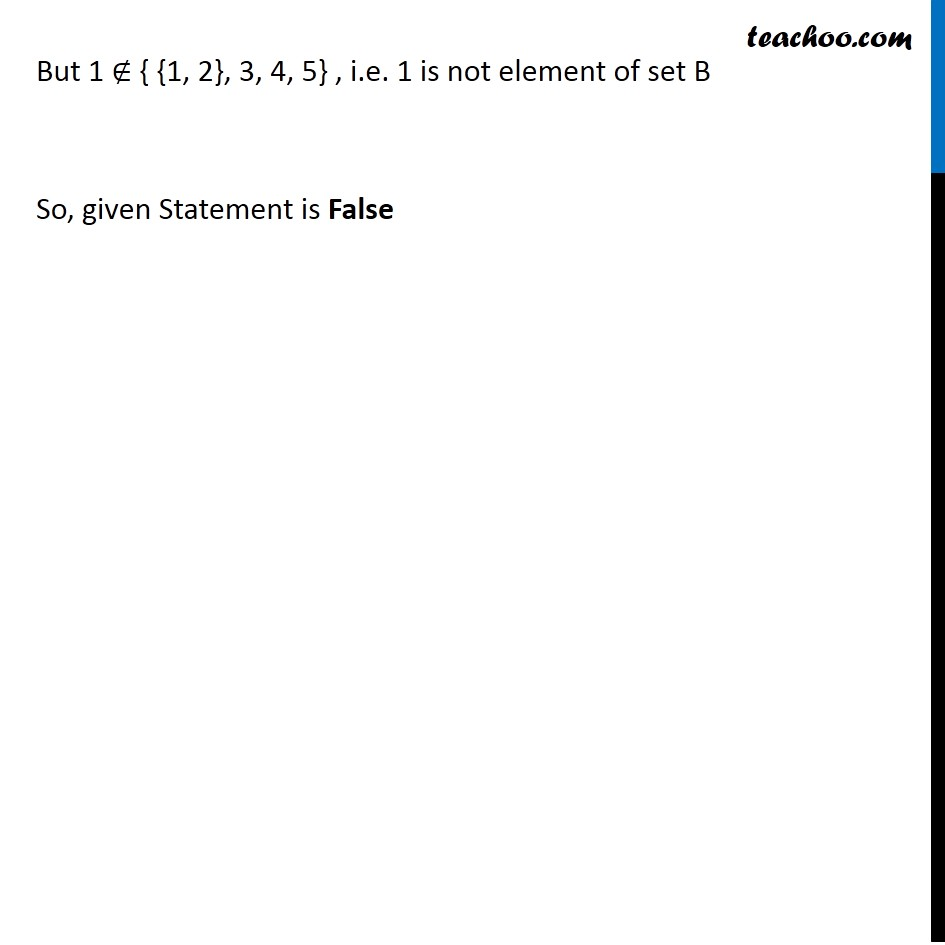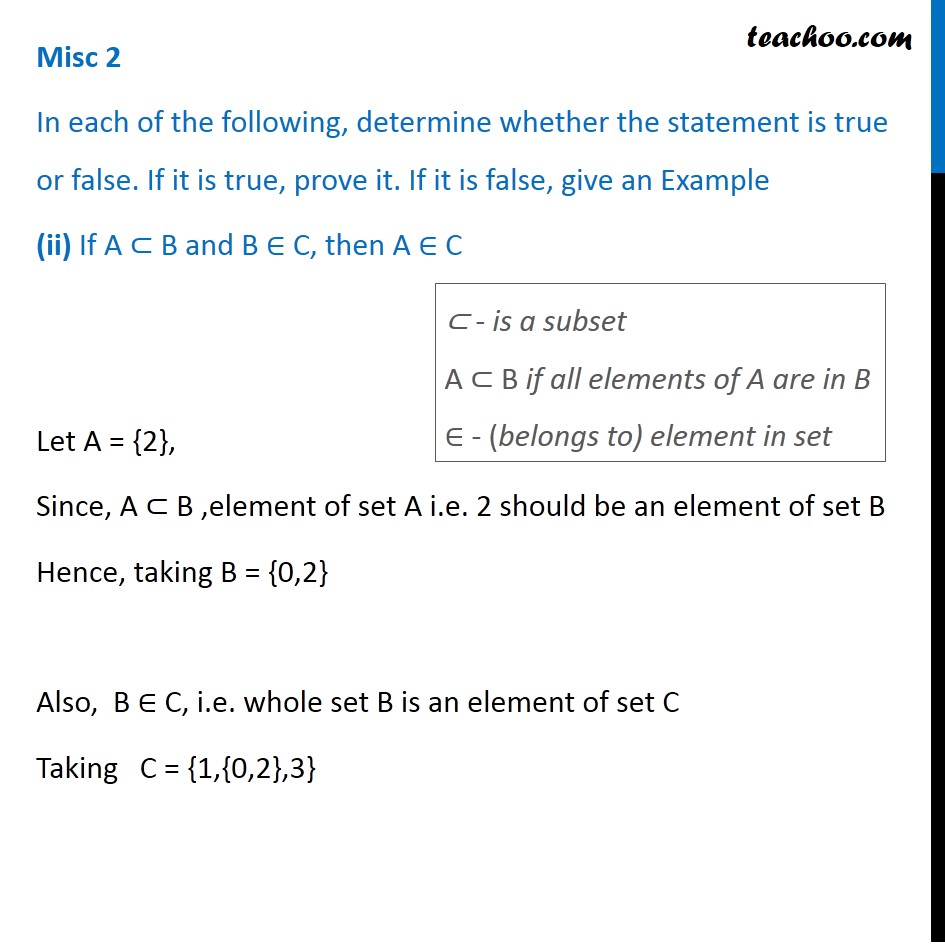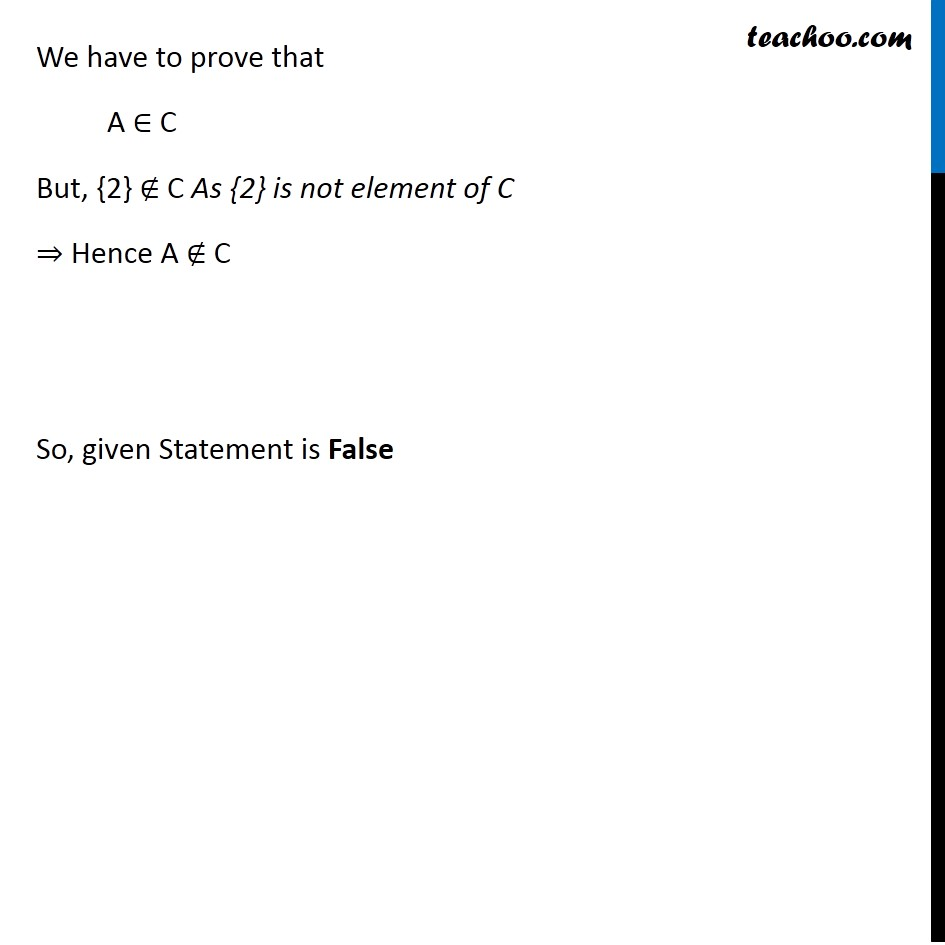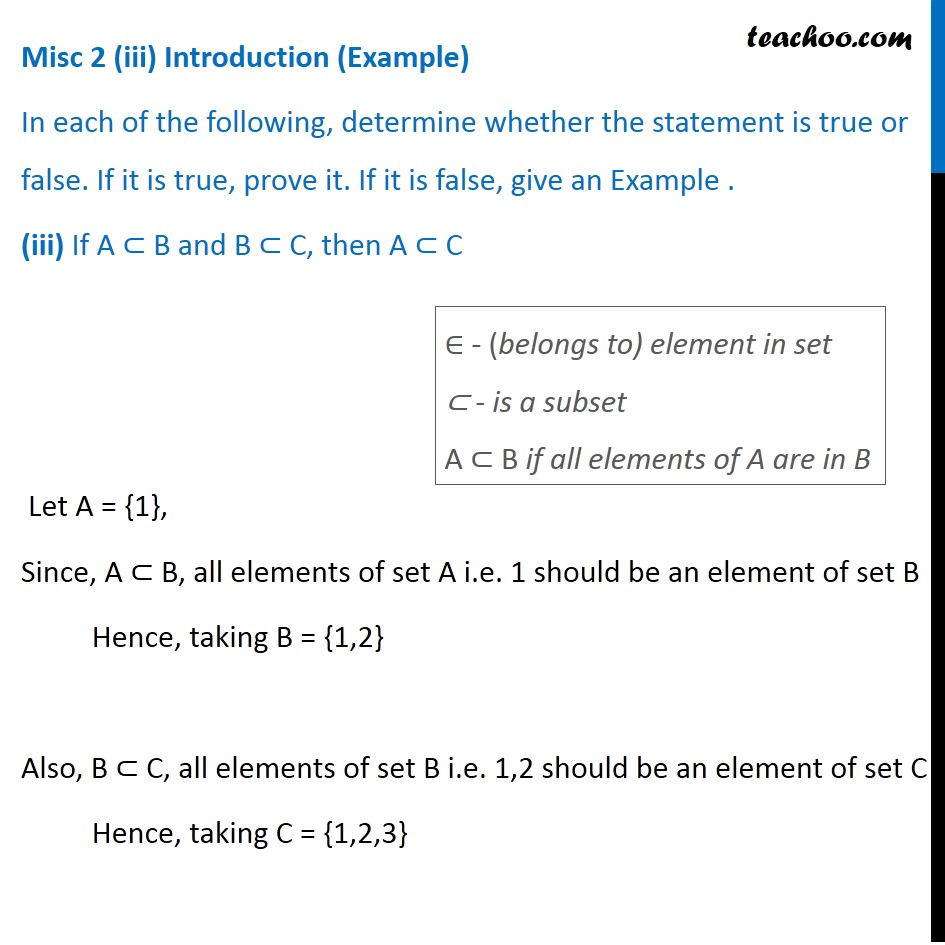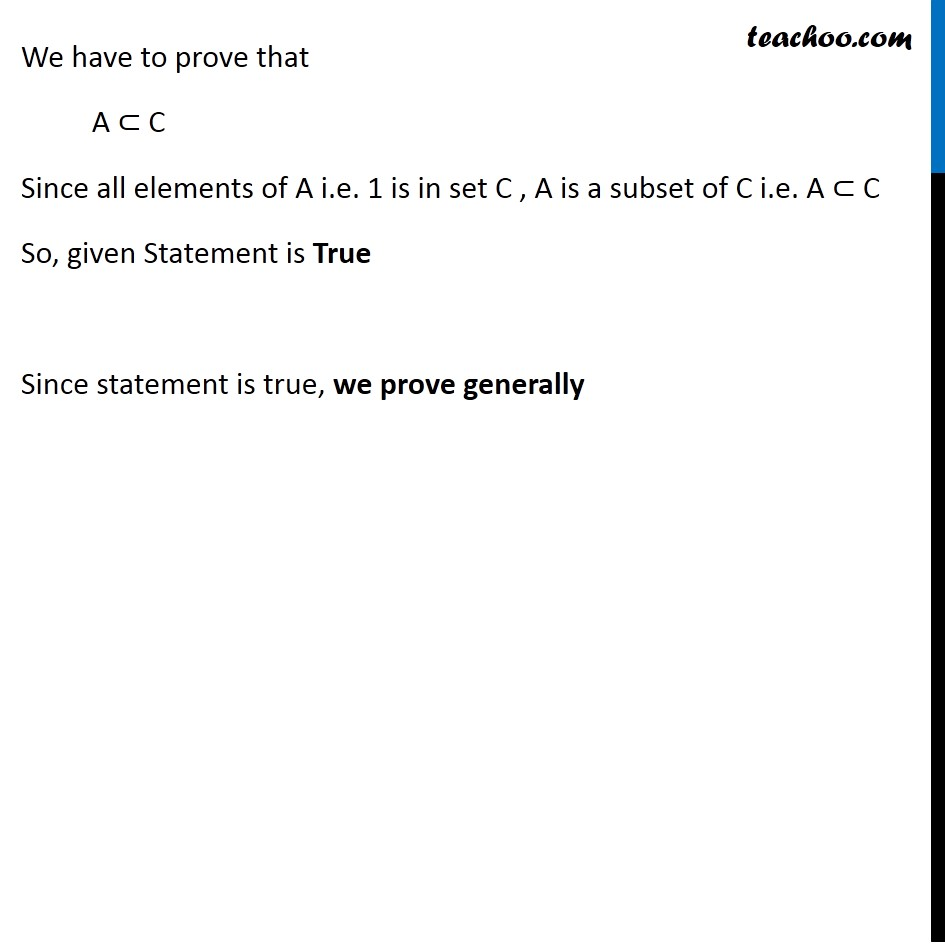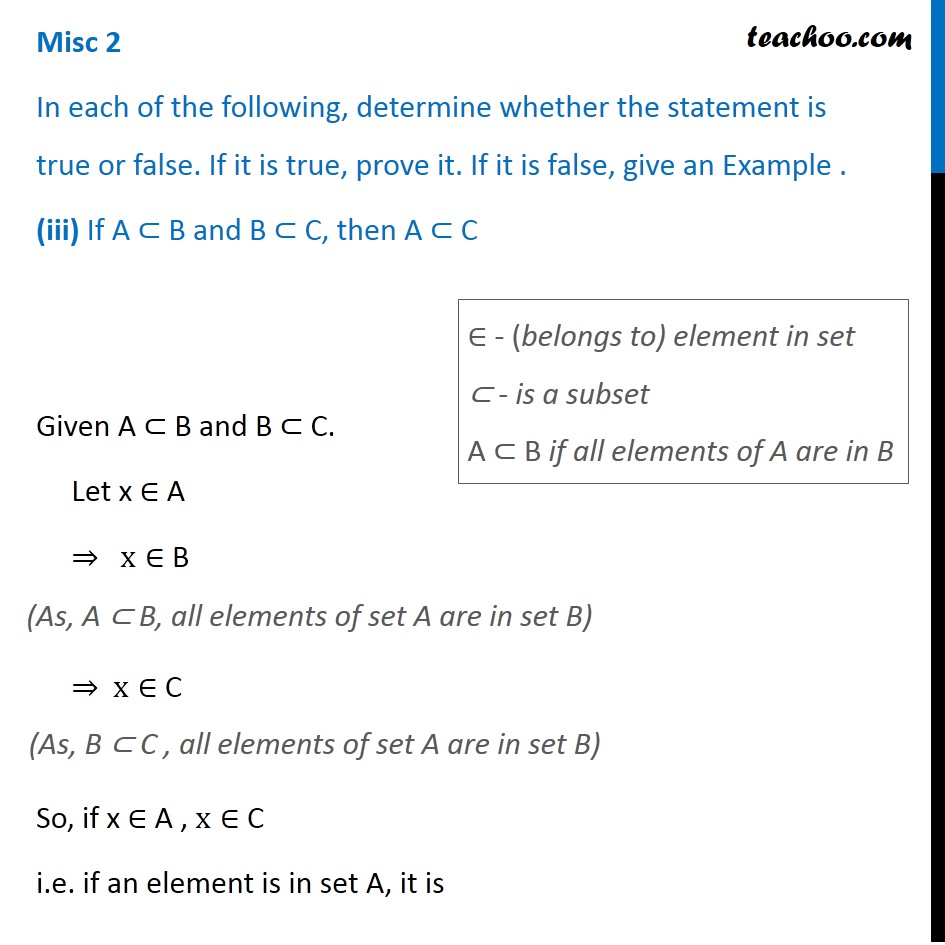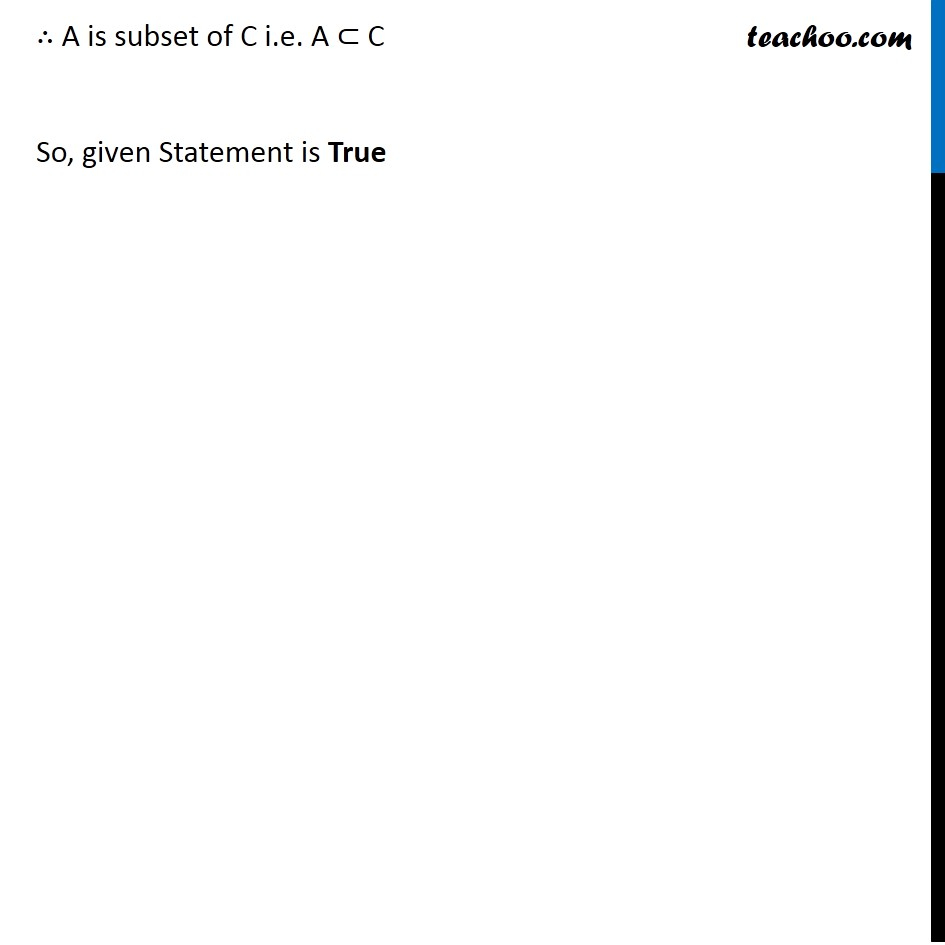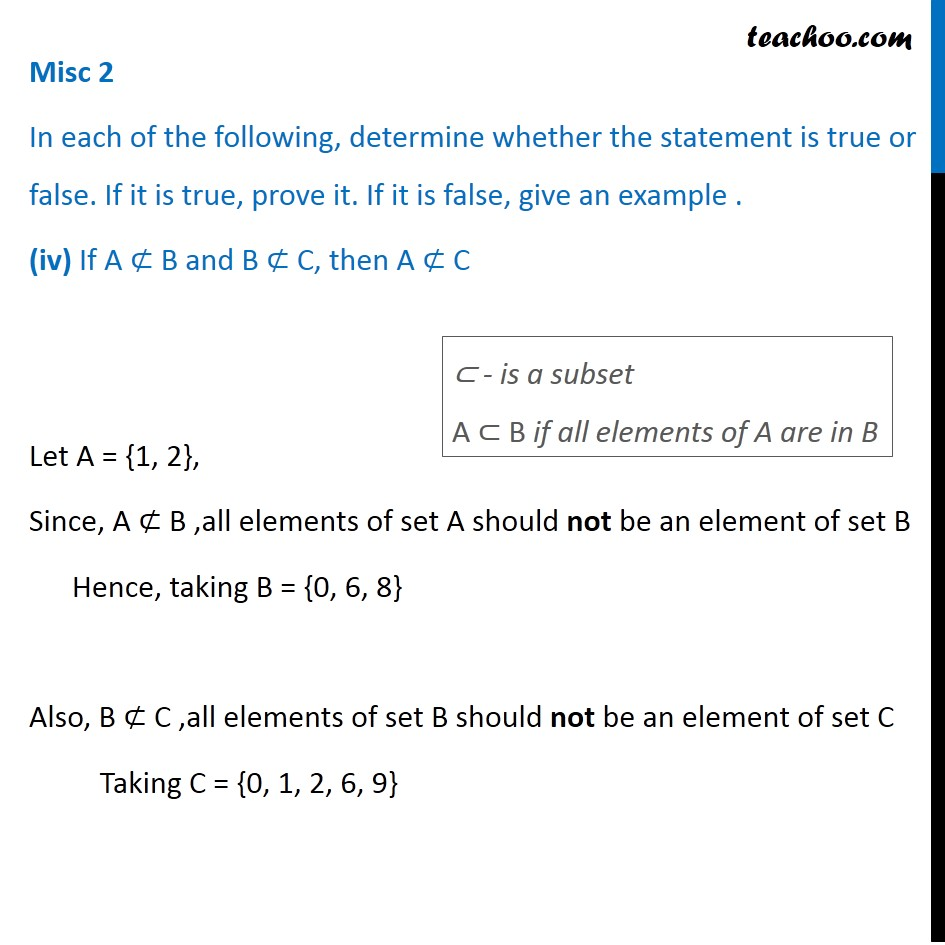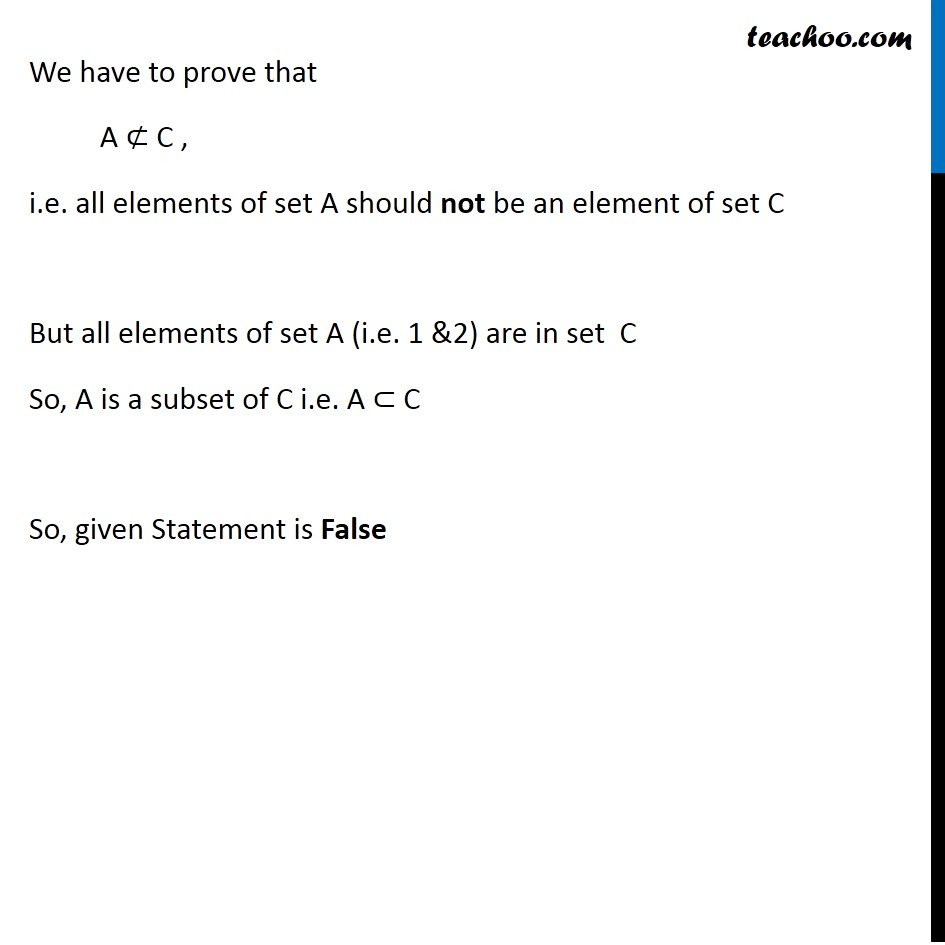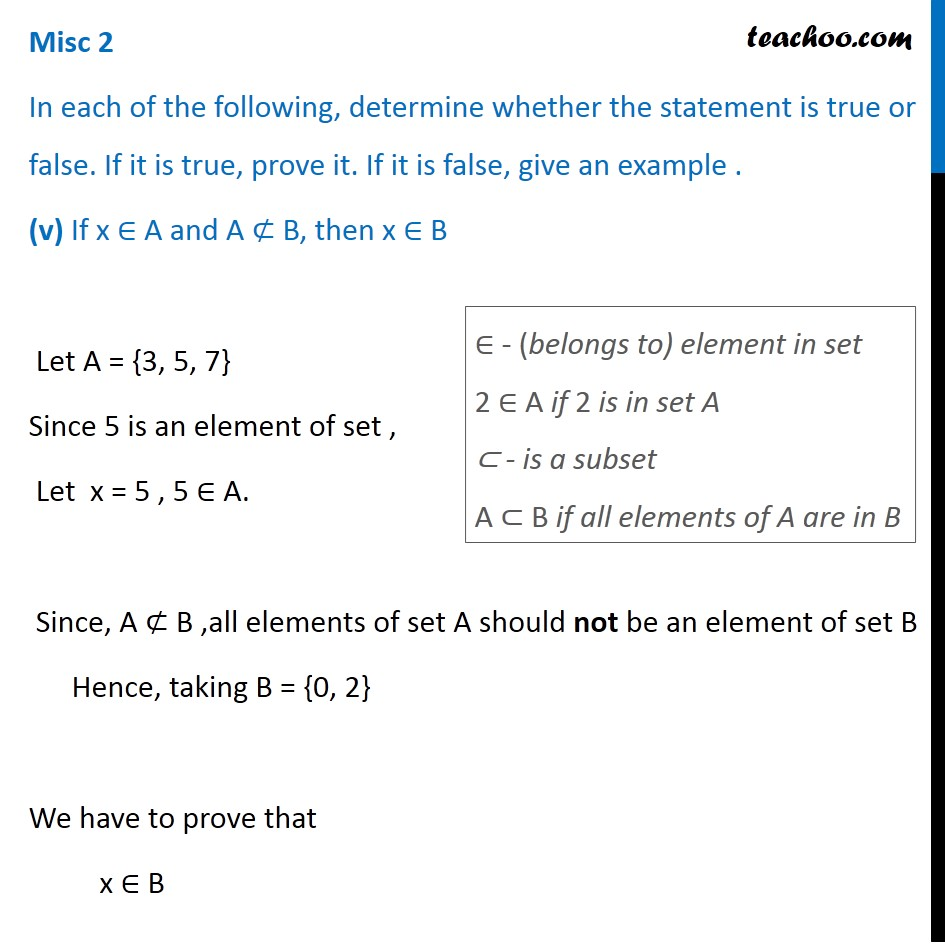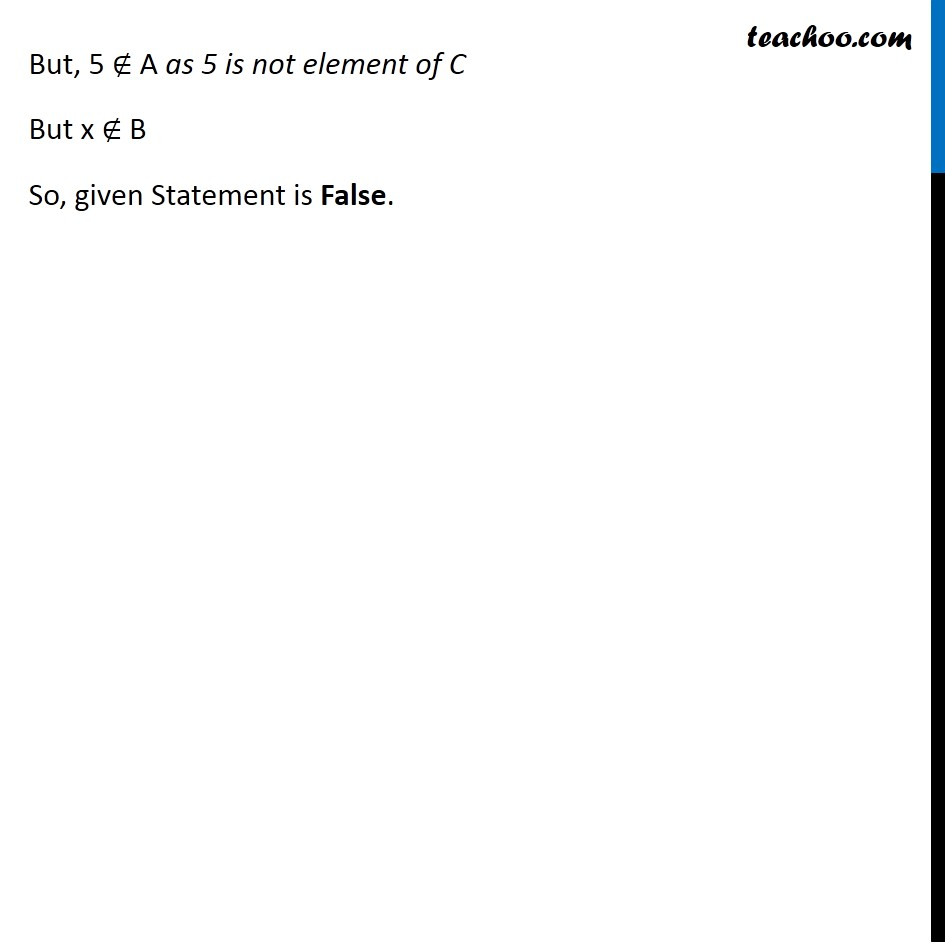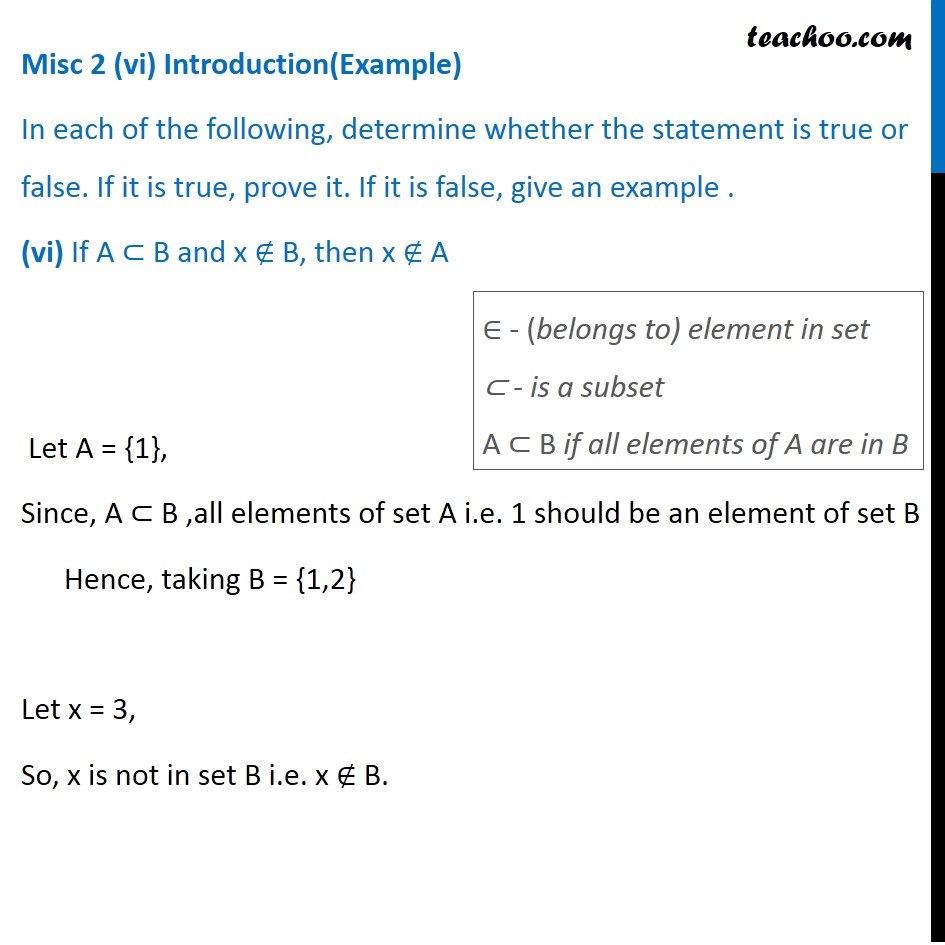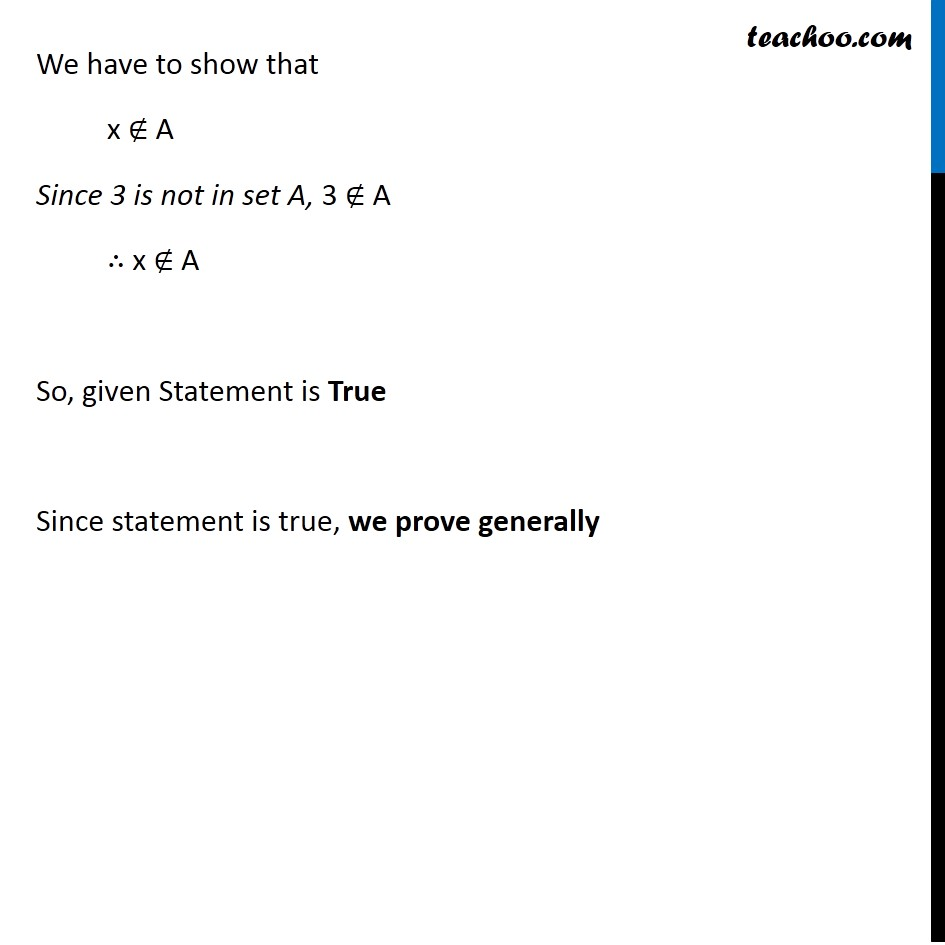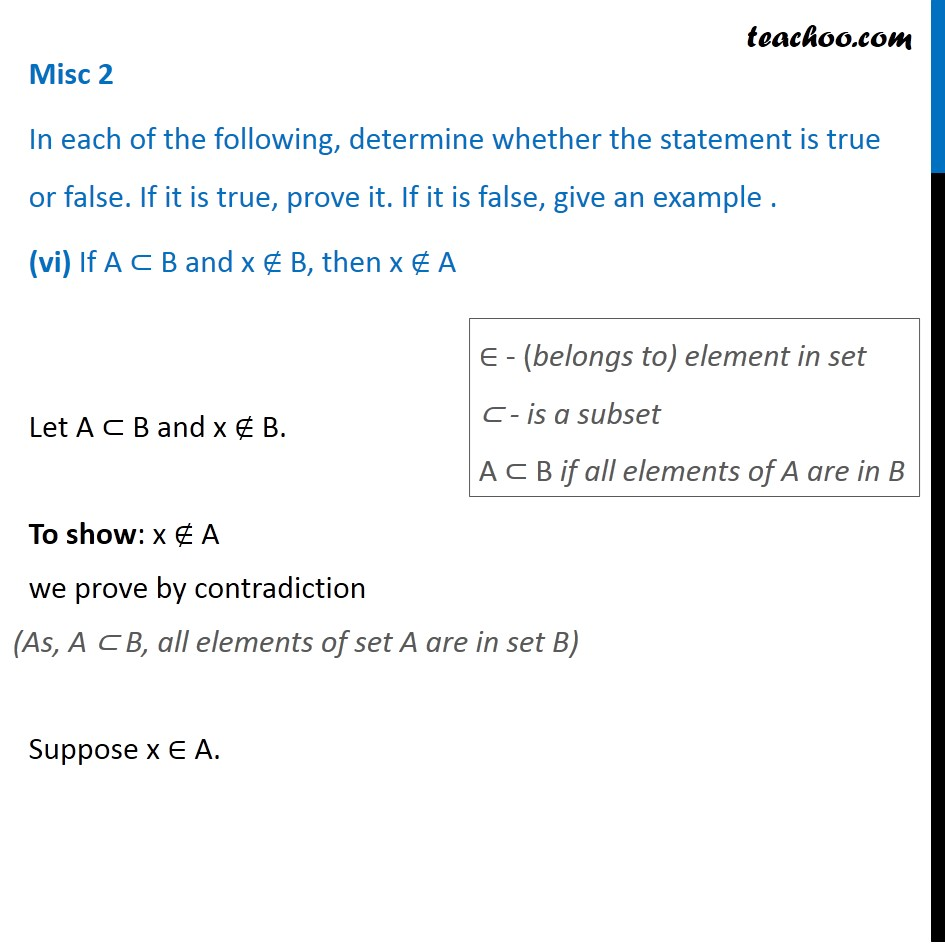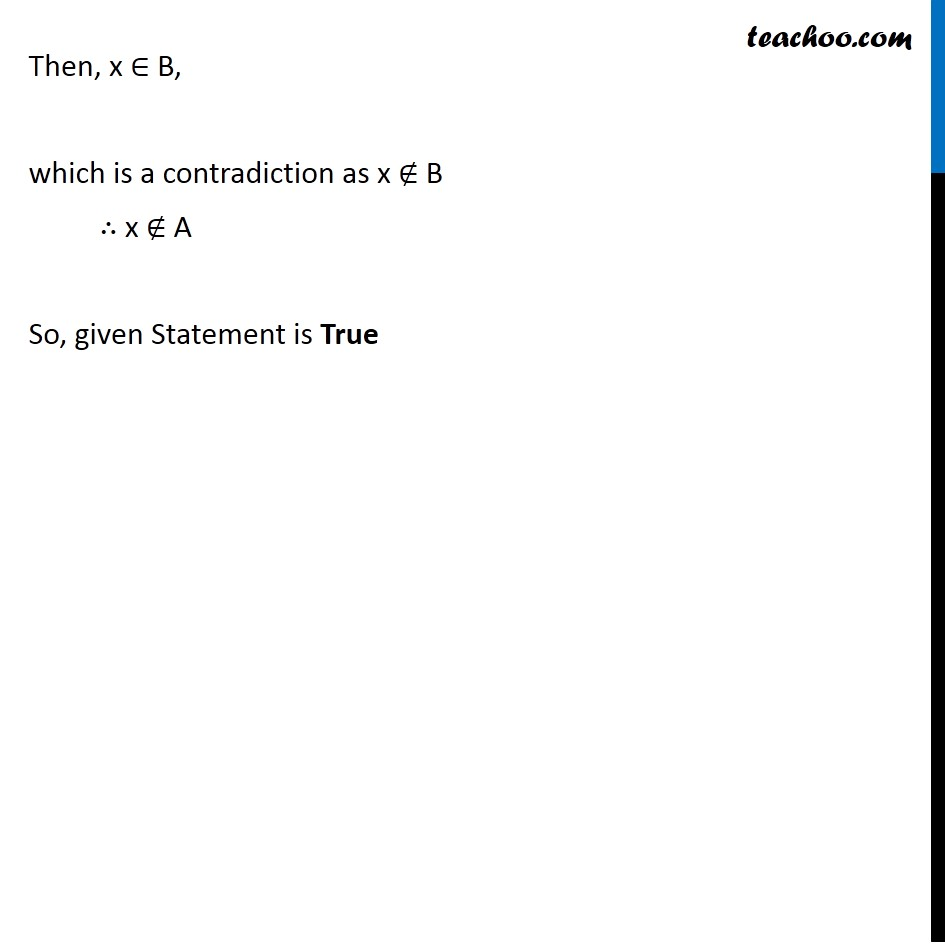1. Chapter 1 Class 11 Sets
2. Serial order wise
3. Miscellaneous

Transcript

Misc 2 In each of the following, determine whether the statement is true or false. If it is true, prove it. If it is false, give an example . (i) If x ∈ A and A ∈ B, then x ∈ B Let A = {1, 2} Since 1 is an element of set , Let x = 1 , 1 ∈ {1,2} . Also, A ∈ B, i.e. whole set A is an element of set B Taking B = { {1, 2}, 3,4,5} We have to prove that x ∈ B ⊂ - is a subset A ⊂ B if all elements of A are in B ∈ - (belongs to) element in set But 1 ∉ { {1, 2}, 3, 4, 5} , i.e. 1 is not element of set B So, given Statement is False Misc 2 In each of the following, determine whether the statement is true or false. If it is true, prove it. If it is false, give an Example (ii) If A ⊂ B and B ∈ C, then A ∈ C Let A = {2}, Since, A ⊂ B ,element of set A i.e. 2 should be an element of set B Hence, taking B = {0,2} Also, B ∈ C, i.e. whole set B is an element of set C Taking C = {1,{0,2},3} ⊂ - is a subset A ⊂ B if all elements of A are in B ∈ - (belongs to) element in set We have to prove that A ∈ C But, {2} ∉ C As {2} is not element of C ⇒ Hence A ∉ C So, given Statement is False Misc 2 (iii) Introduction (Example) In each of the following, determine whether the statement is true or false. If it is true, prove it. If it is false, give an Example . (iii) If A ⊂ B and B ⊂ C, then A ⊂ C Let A = {1}, Since, A ⊂ B, all elements of set A i.e. 1 should be an element of set B Hence, taking B = {1,2} Also, B ⊂ C, all elements of set B i.e. 1,2 should be an element of set C Hence, taking C = {1,2,3} ∈ - (belongs to) element in set ⊂ - is a subset A ⊂ B if all elements of A are in B We have to prove that A ⊂ C Since all elements of A i.e. 1 is in set C , A is a subset of C i.e. A ⊂ C So, given Statement is True Since statement is true, we prove generally Misc 2 In each of the following, determine whether the statement is true or false. If it is true, prove it. If it is false, give an Example . (iii) If A ⊂ B and B ⊂ C, then A ⊂ C Given A ⊂ B and B ⊂ C. Let x ∈ A ⇒ x∈ B ⇒ x∈ C So, if x ∈ A , x∈ C i.e. if an element is in set A, it is ∈ - (belongs to) element in set ⊂ - is a subset A ⊂ B if all elements of A are in B ∴ A is subset of C i.e. A ⊂ C So, given Statement is True Misc 2 In each of the following, determine whether the statement is true or false. If it is true, prove it. If it is false, give an example . (iv) If A ⊄ B and B ⊄ C, then A ⊄ C Let A = {1, 2}, Since, A ⊄ B ,all elements of set A should not be an element of set B Hence, taking B = {0, 6, 8} Also, B ⊄ C ,all elements of set B should not be an element of set C Taking C = {0, 1, 2, 6, 9} ⊂ - is a subset A ⊂ B if all elements of A are in B We have to prove that A ⊄ C , i.e. all elements of set A should not be an element of set C But all elements of set A (i.e. 1 &2) are in set C So, A is a subset of C i.e. A ⊂ C So, given Statement is False Misc 2 In each of the following, determine whether the statement is true or false. If it is true, prove it. If it is false, give an example . (v) If x ∈ A and A ⊄ B, then x ∈ B Let A = {3, 5, 7} Since 5 is an element of set , Let x = 5 , 5 ∈ A. Since, A ⊄ B ,all elements of set A should not be an element of set B Hence, taking B = {0, 2} We have to prove that x ∈ B ∈ - (belongs to) element in set 2 ∈ A if 2 is in set A ⊂ - is a subset A ⊂ B if all elements of A are in B But, 5 ∉ A as 5 is not element of C But x ∉ B So, given Statement is False. Misc 2 (vi) Introduction(Example) In each of the following, determine whether the statement is true or false. If it is true, prove it. If it is false, give an example . (vi) If A ⊂ B and x ∉ B, then x ∉ A Let A = {1}, Since, A ⊂ B ,all elements of set A i.e. 1 should be an element of set B Hence, taking B = {1,2} Let x = 3, So, x is not in set B i.e. x ∉ B. ∈ - (belongs to) element in set ⊂ - is a subset A ⊂ B if all elements of A are in B We have to show that x ∉ A Since 3 is not in set A, 3 ∉ A ∴ x ∉ A So, given Statement is True Since statement is true, we prove generally We have to show that x ∉ A Since 3 is not in set A, 3 ∉ A ∴ x ∉ A So, given Statement is True Since statement is true, we prove generally Misc 2 In each of the following, determine whether the statement is true or false. If it is true, prove it. If it is false, give an example . (vi) If A ⊂ B and x ∉ B, then x ∉ A Let A ⊂ B and x ∉ B. To show: x ∉ A we prove by contradiction Suppose x ∈ A. ∈ - (belongs to) element in set ⊂ - is a subset A ⊂ B if all elements of A are in B Then, x ∈ B, which is a contradiction as x ∉ B ∴ x ∉ A So, given Statement is True

Miscellaneous

Chapter 1 Class 11 Sets
Serial order wise